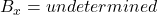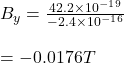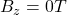## An electron has a velocity of 1.50 km/s (in the positive x direction) and an acceleration of 2.00 ✕ 1012 m/s2 (in the positive z direction)

Question

An electron has a velocity of 1.50 km/s (in the positive x direction) and an acceleration of 2.00 ✕ 1012 m/s2 (in the positive z direction) in uniform electric and magnetic fields. If the electric field has a magnitude of strength of 18.0 N/C (in the positive z direction), determine the following components of the magnetic field. If a component cannot be determined, enter ‘undetermined’.

in progress 0
1 month 2021-07-31T16:44:23+00:00 1 Answers 2 views 0

see explanation

Explanation:

Given that,

velocity of 1.50 km/s = 1.50 × 10³m/s

acceleration of 2.00 ✕ 1012 m/s2

electric field has a magnitude of strength of 18.0 N/C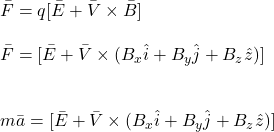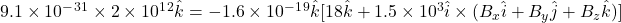$$42.2 \times 10^-^1^9 \hat k = -2.4 \times 10^1^6B_y \hat k + 2.4 \times 10 ^1^6 \hat j B_z\\$$